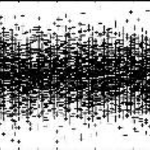Community Profile# Youssef Khmou

DISCLAIMER: Advices or Opinions posted are from author , and do not reflect that of MathWorks.

#### 统计数据

All
••••••••••••#### Content Feed

Distance formula using while loop
In the given formulae, are the variables vectors? if it is the case, then you need to use index in the loop for each variable to...

6 years 前 | 0

The first part of the program is correct, the result is 100 Hz: Fs = 65536; % Sampling Frequency (Hz) Ts = 1/Fs; % Sampli...

6 years 前 | 0

Generating wavepackets in MATLAB
Among the methods for generating wavepackets is linear superposition, considering the spatial dimension x, an elementary complex...

6 years 前 | 1

| 已接受

Randomly select samples from a matrix in proper order
The selection procedure can be performed by ordering the inputs from the smallest to the largets, here is an example, a vector o...

6 years 前 | 0

create a new matrix with elements from different sized matrices
By computing the difference between the two matrices A and B, find the indexes of the entries that equal zero and replace the en...

6 years 前 | 0

J-V characteristic of solar cell
Current density and voltage Charateristic of solar cell using electron diffusion model

8 years 前 | 4 次下载 |Need help on designing plot
defining the variable is the first step : x=0:pi/100:20*pi; using element wise operation : y=x.*sin(x); z=x.*cos(...

8 years 前 | 0

| 已接受

How can i compute inverse of 3-dimensional matrix?
There is misunderstanding between the question and the provided example, according the question, Jhon's answer is correct, three...

8 years 前 | 0

How Fourier Synthesis is performed using Matlab ?
Fourier synthesis is simple application of coefficients summation either numerically or symbolically, Fourier decomposition requ...

8 years 前 | 3

3D Brownian Motion
Fast simulation of three dimensional Brownian motion

8 years 前 | 3 次下载 |Write a function that takes a vector xyz and returns as output two variables
try : function [Mav,Err]=myAver(u) % hint : use sum, length, std,...

8 years 前 | 0

Variable Sampling Frequency Interpolation-hrv
An alternative solution exists using resample(x,p,q) function where the new signal is sampled with p/q times the original sampli...

8 years 前 | 0

Filtering a set of recorded data
The important part of the problem is the implementation of MA filter, here is an example of 6 points window, fs=80; t=0:1...

8 years 前 | 0

Trouble with Envelope Functions
try the following basic solution using Hilbert transform : fs=40; t=0:1/fs:4-1/fs; f=15; y=1.5*sin(2*pi*f*t).*exp(-1.1*...

8 years 前 | 1

how can i plot spherical coordinate?
plotting in the same yields to polar coordinates, try polar(theta,rho); if it is different than the above case, explain ...

8 years 前 | 0

Matlab - FFT/PSD Problem for Preemphasis
I think the first question is not well interpreted, maybe it consists of generating noisy version of y instead of white noise, i...

8 years 前 | 0

Matlab - FFT/PSD Problem for Preemphasis
When using the reverse fft function, we take the real part, in one of the equations H.*G is not necessary since G=H.^-1, another...

8 years 前 | 0

how to compare histograms
Many approaches exist for this purpose, the easiest way is the root mean square error metric, the minimum value means best match...

8 years 前 | 0

Filter of sin component of sound signal
Concerning the equation of y, can you explain its origin. As for the error, initial condition must be set : y=zeros(size(x))...

8 years 前 | 0

Hi all. I need to plot a Fast Fourier Transform(FFT) of a sinc function
Let us consider non causal function, we can use built in function as follows : Fs=42;Ts=1/Fs; t=-1:Ts:40*Ts-Ts; f=5; y...

8 years 前 | 1

How to derivate a vector
additionally to the above answers, the simplest way to evaluate the polynomial is via anonymous function : f=@(x) x.^2+x-1...

8 years 前 | 0

Preventing all [x,y] figures appearing when running a plot of a circle
Adding semi column (;) for all instructions inside the function will prevent the printing of values.

8 years 前 | 0

How can i set (f) = (-60/(s^2+5s+48.5))?
Element wise operation fixes the error : f=-60./(s.^2+5*s+48.5);

8 years 前 | 0

Trying to make a function for summing up 3 numbers. Explaination below
This function works for a vector of length n, with second logical output according to the description : function [Logical...

8 years 前 | 0

How to I get a threshold value from looking at histogram
Otsu's method exists as built in function with name *graythresh*, here is an example taken from Mathworks help page : I = i...

8 years 前 | 1

Matlab analytical FT and FFT comparison
numerical fft requires a shift if we want to visualize the spectrum with both negative and positive frequencies, scaling problem...

8 years 前 | 0

Trying to make a function for summing up 3 numbers. Explaination below
the following answer is an alternative approach without using 'getdigits' function : function [C,y]=sum3(x) h=int2st...

8 years 前 | 0

| 已接受

Driven Damped Pendulum Axes Question
The implementation is acceptable, the first remark is that visualization commands of results should be outside the loop, otherwi...

9 years 前 | 0

| 已接受

Is 'newplot' the reason why 'image' and 'plot' are no longer working on my computer?
In previous version of software it works correctly, if you have used the name 'image' for your constructed function, rename it. ...

9 years 前 | 0

Adding white gaussian Noise to 1D signals
To produce a unique sequence of white Gaussian noise, you need to reset the sate as follows : x=sin(2*pi*0.2*(0:0.1:10));...

9 years 前 | 0

| 已接受